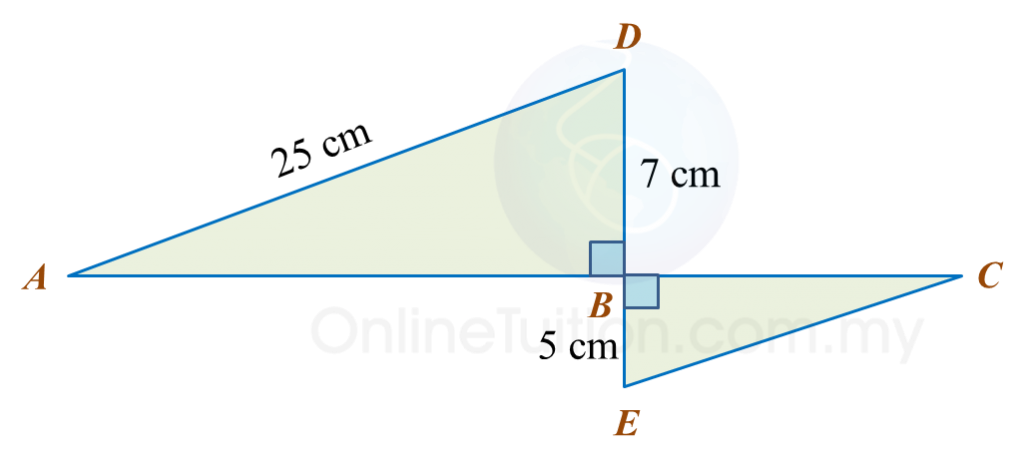# 13.2.2 The Pythagoras’ Theorem, PT3 Focus Practice

 
          
 Question 3:
  In diagram below, ABCH is a square and DEFG is a rectangle. HCDE is a straight line and HC = CD.Find the perimeter, in cm, of the whole diagram.  
 
  

 Solution:    
                     G    H    2      =D    H    2      +D    G    2                     =    24    2      +    7    2                     =576+49                =625           GH=25 cm                      Perimeter of the whole diagram           =12+12+12+26+7+14+25           =108 cm                    
  
 
 
          
 Question 4:
  In the diagram, ABC and EFD are right-angled triangles.Calculate the perimeter, in cm, of the shaded region.  
 
  

 Solution:    
                     D    E    2      =    3    2      +    4    2                     =9+16                =25           DE=       25                   =5 cm                      A    C    2      =    7    2      +    24    2                     =49+576                =625           AC=       625                   =25 cm                      Perimeter of the shaded region           =24+7+(       255   )+3+4           =58 cm                    
  
 
 
          
 Question 5:
  In the diagram, ABD and BCE are right-angled triangles. ABC and DBE are straight lines. The length of AB is twice the length of BC.Calculate the length, in cm, of CE.  
 
  

 Solution:    
                     A    B    2      =    25    2          7    2                     =62549                =576           AB=       576                   =24 cm           BC=24 cm÷2              =12 cm           C    E    2      =    5    2      +    12    2                     =25+144                =169           CE=       169                   =13 cm